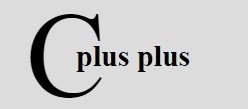# C++ Access Structure Member

« Previous Tutorial Next Tutorial »

In C++, once a structure variable has been defined, its member can be accessed through the use of dot (.) operator. For example, the following code fragment assigns 1740 to year element of birth_date structure variable declared earlier:

`birth_date.year = 1740 ;`

## Syntax to Access Structure Member in C++

The structure variable name followed by a period or dot (.) and the element name references to that individual structure element. The syntax to access structure element is shown here:

`structure-name.element-name`

Remember that the first component or element of an expression involving the dot (.) operator is the name of specific structure variable (birth_date in this case), not the name of structure specifier (date).

The structure members are treated just like other variables. So, to print year of birth_date, you can simply write:

`cout << birth_date.year ;`

In same fashion, to read day, month and year of joining_date, you can simply write :

`cin >> joining_date.day >> joining_date.month >> joining_date.year ;`

## C++ Access Structure Members Example

Here is an example, demonstrating how to access members of a structure in C++

```/* C++ Access Structure Member */

#include<iostream.h>
#include<conio.h>

struct st
{
int a;    // structure member
int b;    // structure member
int sum;  // structure member
}st_var;         // structure variable

void main()
{
clrscr();
cout<<"Enter any two number:\n";
// accessing structure member a and b
cin>>st_var.a>>st_var.b;
// accessing structure member sum, a, and b
st_var.sum = st_var.a + st_var.b;
// accessing structure member sum
cout<<"\nSum of the two number is "<<st_var.sum;
getch();
}```

Here is the sample run of this C++ program:

C++ Online Test

« Previous Tutorial Next Tutorial »